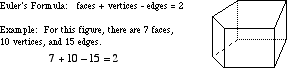index: click on a letter A B C D E F G H I J K L M N O P Q R S T U V W X Y Z A to Z index index: subject areas numbers & symbols sets, logic, proofs geometry algebra trigonometry advanced algebra & pre-calculus calculus advanced topics probability & statistics real world applications multimedia entrieswww.mathwords.com about mathwords website feedback

 Euler's Formula (Polyhedra) The equation below: (number of faces) + (number of vertices) – (number of edges) = 2 This formula is true for all convex polyhedra as well as many types of concave polyhedra. Note: Euler is pronounced "Oiler".